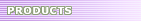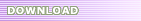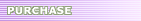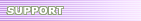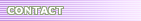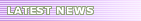# Ellemtel C++ Style and Rules - Pointers and References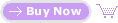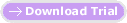12. Pointers and References Rule 42Do not compare a pointer to NULL or assign NULL to a pointer; use 0 instead. [An intensive debate about this has been raging in the news group comp.lang.c++. Future changes in this recommendation may occur.] Rec. 48Pointers to pointers should whenever possible be avoided. Rec. 49Use a `typedef` to simplify program syntax when declaring function pointers. According to the ANSI-C standard, `NULL` is defined either as `(void*)0` or as `0`. If this definition remains in ANSI-C++, problems may arise. If NULL is defined to have the type `void*`, it cannot be assigned an arbitrary pointer without an explicit type conversion. For this reason, we recommend comparisons with 0 at least until the ANSI-C++ committee has made a decision. Pointers to pointers normally ought not be used. Instead, a class should be declared, which has a member variable of the pointer type. This improves the readability of the code and encourages data abstraction. By improving the readability of code, the probability of failure is reduced. One exception to this rule is represented by functions which provide interfaces to other languages (such as C). These are likely to only allow pre-defined data types to be used as arguments in the interface, in which case pointers to pointers are needed. Another example is the second argument to the `main` function, which must have the type `char*[]`. [This is equivalent to `char**`.] A function which changes the value of a pointer that is provided as an argument, should declare the argument as having the type reference to pointer (e.g. `char*&`). See Rule 42! `typedef` is a good way of making code more easily maintainable and portable. See chapter 18.1, Port.Rec.1. Another reason to use `typedef` is that the readability of the code is improved. If pointers to functions are used, the resulting code can be almost unreadable. By making a type declaration for the function type, this is avoided. Function pointers can be used as ordinary functions; they do not need to be dereferenced. [See Example 46.] Exception to Rule 42No exceptions. Example 45: Different comparisons of pointers ``` char* sp = new char; if ( !sp ) cout << "New failed!" << endl; // No! if ( sp == 0 ) cout << "New failed!" << endl; // Best if ( sp == NULL ) cout << "New failed!" << endl; // ERROR sometimes !!! ``` Example 46: Pointers to pointers are often unnecessary [This example is, in part, taken from The C++ Programming Language, Second Edition.] ``` #include void print_mij(int** m, int dim1, int dim2) { for (int i = 0; i < dim1; i++) { for (int j = 0; j < dim2; j++ ) cout << " " << ((int*)m)[i*dim2+j]; cout << endl; } } // Could be written as: class Int_Matrix { public: Int_Matrix(int dim1, int dim2); int value(int,int) const; int dim1() const; int dim2() const; // .. }; void print_Mij(Int_Matrix m) { for (int i = 0; i < m.dim1(); i++) { for (int j = 0; j < m.dim2(); j++ ) cout << " " << m.value(i,j); cout << endl; } } ``` Example 47: Complicated declarations ``` // func1 is a function: int -> (function : const char* -> int) // i.e. a function having one argument of type int and returning // a pointer to a function having one argument of type const char* // and returning an int. int (*func1(int))(const char*); // func1 of the same type as func2 typedef int FTYPE(const char*); FTYPE* func2(int); int (*(*func1p)(int))(const char*) = func2; // Realistic example from signal.h void (*signal(int,void (*)(int)))(int); ``` Example 48: Syntax simplification of function pointers using a `typedef` ``` #include // Ordinary messy way of declaring pointers to functions: // double ( *mathFunc ) ( double ) = sqrt; // With a typedef, life is filled with happiness (chinese proverb): typedef double MathFuncType( double ); MathFuncType* mathFunc = sqrt; void main() { // You can invoke the funktion in an easy or complicated way double returnValue1 = mathFunc( 23.0 ); // Easy way double returnValue2 = ( *mathFunc )( 23.0 ); // No! Correct, but complicated } ```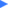Working with a team who put C++ pointer symbols in strange way?  Try C++ Code Beautifier to beautify your C++ source code rapidly.